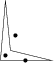# Chapter 162D Polygons

 16.1 Introduction 16.2 Example

## 16.1   Introduction

A polygon is a closed chain of edges. Several algorithms are available for polygons. For some of those algorithms, it is necessary that the polygon is simple. A polygon is simple if edges don't intersect, except consecutive edges, which intersect in their common vertex.

The following algorithms are available:

• find the leftmost, rightmost, topmost and bottommost vertex.
• compute the (signed) area.
• check if a polygon is simple.
• check if a polygon is convex.
• find the orientation (clockwise or counterclockwise)
• check if a point lies inside a polygon.
All those operations take two forward iterators as parameters in order to describe the polygon. These parameters have a point type as value type.

The type Polygon_2 can be used to represent polygons. Polygons are dynamic. Vertices can be modified, inserted and erased. They provide the algorithms described above as member functions. Moreover, they provide ways of iterating over the vertices and edges.

Currently, the Polygon_2 class is a nice wrapper around a container of points, but little more. Especially, computed values are not cached. That is, when the is_simple() member function is called twice or more, the result is computed each time anew.

## 16.2   Example

The following code fragment creates a polygon and does some checks.

```File: examples/Polygon/Polygon.cpp
```
```#include <CGAL/Exact_predicates_inexact_constructions_kernel.h>
#include <CGAL/Polygon_2.h>
#include <iostream>

typedef CGAL::Exact_predicates_inexact_constructions_kernel K;
typedef K::Point_2 Point;
typedef CGAL::Polygon_2<K> Polygon_2;
using std::cout; using std::endl;

int main()
{
Point points[] = { Point(0,0), Point(5.1,0), Point(1,1), Point(0.5,6)};
Polygon_2 pgn(points, points+4);

// check if the polygon is simple.
cout << "The polygon is " <<
(pgn.is_simple() ? "" : "not ") << "simple." << endl;

// check if the polygon is convex
cout << "The polygon is " <<
(pgn.is_convex() ? "" : "not ") << "convex." << endl;

return 0;
}
```Figure 16.1:  A polygon and some points

```File: examples/Polygon/polygon_algorithms.cpp
```
```#include <CGAL/Exact_predicates_inexact_constructions_kernel.h>
#include <CGAL/Polygon_2_algorithms.h>
#include <iostream>

typedef CGAL::Exact_predicates_inexact_constructions_kernel K;
typedef K::Point_2 Point;
using std::cout; using std::endl;

void check_inside(Point pt, Point *pgn_begin, Point *pgn_end, K traits)
{
cout << "The point " << pt;
switch(CGAL::bounded_side_2(pgn_begin, pgn_end,pt, traits)) {
case CGAL::ON_BOUNDED_SIDE :
cout << " is inside the polygon.\n";
break;
case CGAL::ON_BOUNDARY:
cout << " is on the polygon boundary.\n";
break;
case CGAL::ON_UNBOUNDED_SIDE:
cout << " is outside the polygon.\n";
break;
}
}

int main()
{
Point points[] = { Point(0,0), Point(5.1,0), Point(1,1), Point(0.5,6)};

// check if the polygon is simple.
cout << "The polygon is "
<< (CGAL::is_simple_2(points, points+4, K()) ? "" : "not ")
<< "simple." << endl;

check_inside(Point(0.5, 0.5), points, points+4, K());
check_inside(Point(1.5, 2.5), points, points+4, K());
check_inside(Point(2.5, 0), points, points+4, K());

return 0;
}
```